@Pigmon 2016-05-30T17:27:43.000000Z 字数 6650 阅读 135

# 文献报告_袁胜_2016M8009073008

上课

## 论文题目：

Sparse Color Interest Points for Image Retrieval and Object Categorization
《针对图像检索和物体分类的稀疏颜色兴趣点提取方法》

## 作者

Julian Stöttinger, Allan Hanbury, Nicu Sebe, Senior Member, IEEE, and Theo Gevers, Member, IEEE

## 期刊

IEEE TRANSACTIONS ON IMAGE PROCESSING, VOL. 21, NO. 5, MAY 2012

## 摘要

作者首先强调了兴趣点在图像处理和计算机视觉中的重要性。以及，通常兴趣点的产生是基于亮度（luminance-based）的。但如果把颜色信息应用在产生兴趣点的算法中，会增强兴趣点的可区分性（distinctiveness），并且在图像匹配的过程中会显著的提高效率。

• 在稀疏图像表示中使用基于颜色的兴趣点。
• 介绍了为了降低复杂图像环境的敏感度而应用的光照不变兴趣点。
• 基于显著性特征选取以及颜色呈现几率的统计信息而产生的颜色增强点。
• 通过对图像的主要组件分析的尺度选择方法对兴趣点进行鲁棒的缩放。

通过放大实验，论文提及的颜色兴趣点检测器比通常的基于亮度的兴趣点算法有更高的可再现性（repeatability）。
在图像检索实验中，一组数量降低并可预测的颜色特征表现出了比目前主流的兴趣点方法拥有更高的效率。

## I 介绍

作者再次强调了兴趣点在图像处理和计算机视觉中的重要性，以及当前的兴趣点提取算法都是基于亮度的。并再次的强调了颜色在兴趣点提取中的重要性（颜色有更好的区分性，颜色在图像匹配中很重要，颜色是视觉中很重要的刺激因素）
目前的物体识别研究大多针对增加兴趣点，多检测器组合等增加算法复杂度的方向，但随着图像和视频数据的数量不断的加速增长，这样的方向显然是不合适的。应该想办法减少特征数量，让工作量控制在可预见的范围内。

• 基于颜色且尺度无关（scale invariant）的多通道Harris角点检测器。
• 混合局部尺度无关特征的感知颜色空间。
• 图像匹配过程的各个步骤都因为降低了局部特征的数量而得到效率的提升。
• 可以处理更高维度的数据。

## II 相关工作

### A. 图像检索和物体分类的通常步骤

1. 特征提取 2. 描述子 3. 聚类 4. 匹配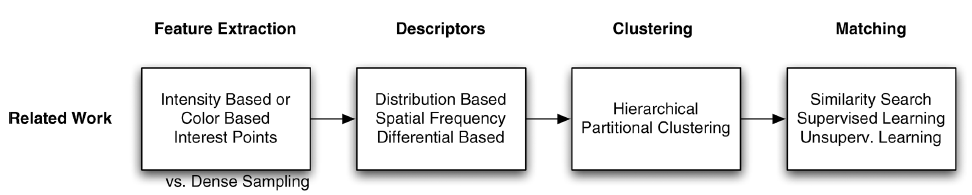### B. 兴趣点

作者在这一节列举了多个兴趣点提取的方法，并进行了对比。另外说明了论文中的基于颜色的兴趣点提取方法是基于$Harris–Laplacian$算法。

## III 颜色兴趣点检测（COLOR INTEREST POINT DETECTION）

在这一章里，作者介绍了一个多通道的Harris角检测方法和一个尺度选择（Scale Selection）方法。这些方法可适应任何颜色空间（Color Space）。
之后的内容，首先讨论了不同的颜色空间，之后是基于颜色的兴趣点检测，最后是尺度选择方法。

### A. 颜色空间（Color Spaces）

首先是基于RGB色彩空间变换而来的OCS（opponent color space）颜色空间。变换使用了可旋转的色度（chromaticity）坐标轴和不同的归一化（normalization），提供镜面（specular）不变性。公式为：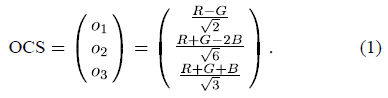之后提出，根据这个OCS模型，通过极坐标变换而来的HSI色彩空间，公式为：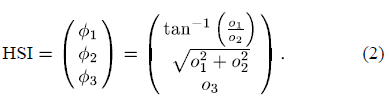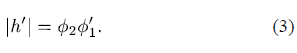$p(v)$为颜色出现的概率，$\nu(v)$为描述子中$p(v)$对应的信息量（information contents）。其中$\nu(v)=-log(p(v))$

颜色增强（color boosting）的关键是，增强那些显著性高但出现频率低的颜色。普遍来说，具有相同向量范数（vector norm）的颜色向量的梯度，在显著性方程中应具有相同的强度（impact）。
作者的目的是，找到一个颜色增强（color boosting）函数$g$，使得具有相同信息量（information contents）的颜色向量，在显著性函数（saliency function）中，具有相同的强度（impact）。

在位置$x$处的显著性$s$，有下列公式给出：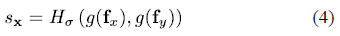显著性增强函数的公式如下：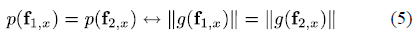变换推导自一个将颜色在3个方向上的分布模拟成一个3D表面的函数，这样可以把这种分布近似的表示成一个椭球体。椭球体中最长的轴代表亮度（luminance）分量，颜色向量的其他2个轴是可旋转的，这样可以让它们跟椭球体中其他两个轴对齐。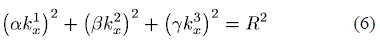为了得到公式（5）中的变换量，椭球体被缩放成一个球体，这样，具有相同显著性的向量的长度就相同了。这样，函数$g$的定义为以下公式：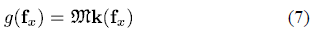### B. 基于颜色的兴趣点（Color-Based Interest Points）

矩阵$M$的二阶矩是描述一个单通道图像$I$中位置$x$的本地邻域的梯度分布的结构张量。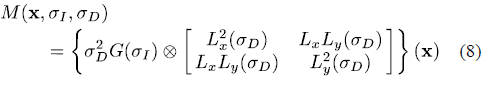广泛的说，二阶矩矩阵可以根据RGB颜色空间的变换计算得出。第一步是决定RGB系统中每个组件的梯度，梯度变换到预想的颜色系统。通过对变换的梯度进行一系列的乘法和加法运算，二阶矩矩阵的各个分量就计算出来了。
用符号化的方式表述，一个颜色空间由它的$n$个组件$[c_1...c_n]^T$构成，那么矩阵M的各个分量以通用性的公式表述如下：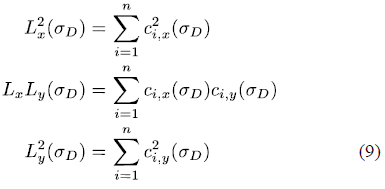众多的实验表明，令$3\sigma_D=\sigma_I$会获得最好的表现。

基于$M$的特征值，Harris能量$\mathfrak{C}$通过如下公式计算：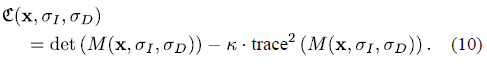这样会导致固定位置的鲁棒噪音，尺度增加到$\sqrt 2$，以及在任意颜色空间的平移和旋转。对很多计算机视觉任务来说，在这种情况下很难保证获取尺度不变的特征。所以，在下一阶段，作者又提出了一个在任意颜色空间，为局部特征来建立基于显著性不变的特征尺度（characteristic scale）的理论模型。

### C. 基于颜色的尺度检测（Color-Based Scale Detection）

文中的尺度选择是通过一个单通道显著性图像$\widehat{I}$来实现的。概念类似于特征图像（eigenimages），区别是没有图像模板（image templates）的训练集，取而代之的是颜色通道信息被当作了颜色分布的模板。

令颜色空间$C$中的图像$I_c={[f_1,...f_m]}$，其中$f_i=[c_1...c_n]$为由$n$个颜色分量组成的颜色向量，颜色的每个组件都是归一化且具有0均值。$Q$$I_c$的协方差矩阵；$e_i$$Q$的特征向量；$\lambda_i$$e_i$的特征值。因为$n\ll m$，所以$n$个特征向量可以很容易通过单值分解得到。特征向量根据对应特征值递减的顺序排列。
$\widehat{I}=[\widehat{f_1}...\widehat{f_m}]$ 其中$\widehat{f_i}=f_ie_1$        (11)

$LoG$（Laplacian of Gaussian）给出高斯斑点中的最大值。通过$LoG$的尺度可以得到斑点的尺寸。也就是说，其目的是为了得到局部结构的特征尺度（characteristic scale）。为了更鲁棒的处理噪音，我们定义尺度$\sigma$，位置$x$上的$\Lambda_{x,\sigma}$为：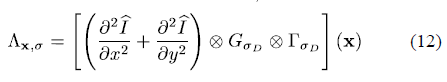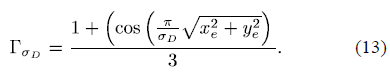相对于高斯核$G$，用这个核进行卷积可以得到更加平滑的边缘，以及可以处理更大型结构的检测操作。
为了提高效率，$\Lambda$可以近似的计算为：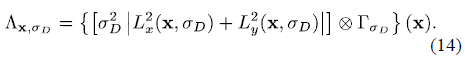角的位置会随着尺度变化而变化，尺度遍历中相邻的变化越小，得到的位置就越精确。Harris检测中把尺度变化因子鲁棒的定义为$t=\sqrt{2}$，因此这个因子值在很多情况下被当作标准使用。Harris函数中的尺度空间通过在变化的$\sigma$下计算Harris能量而得到。不同尺度检查的数量直接影响到执行效率。每一步必须负责自己的运算（同时也是不相关的，也就有了并行计算的可能性），执行时间会随着核的尺寸增加而增加。

令尺度等级$l_s=1,2...$，因子$t$的取值从$1.2$$\sqrt2$，尺度为$t^s \sigma$，进行Harris能量的计算。在Harris能量和$LoG$都达到最大值的时候，可以计算出一个可能范围内潜在的特征尺度：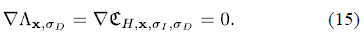这样就计算出了各个位置对应的尺度，但每个位置可能有多个候选的尺度，而每个候选的尺度都要考虑进来。这样就得到了$\Lambda_{x,\sigma}$检测的最大结构尺寸的近似值。通过选定常量$\sigma$$t$，函数$\widehat{E_x}$给出了$\mathfrak{C}_{H,s,\sigma_I,\sigma_D}$的本地最大值，而$\widehat{\Lambda}_X$给出了$\Lambda_{X,\sigma_D}$本地最大值的最大尺度。也就是说，$\widehat{E_x}$$3t^{arg max(\widehat{\Lambda})}\sigma_D$定义了所有的候选兴趣点和其相应的区域大小。多通道Harris能量$\mathfrak{C}_H$是一个显著性属性，可以用来选择兴趣点。

### D. 光照不变点（Light-Invariant Points）

为了从任意彩色图像得到不变点集，需要把输入图像转换成亮度不变图像$I_c=[f_1...f_m]$，其中$f_i=[\phi_1,\phi_2]$。HSI颜色空间中代表亮度的组件$\phi_3$被删除。$c_{1,[x|y]}$通过公式（3）中得到的$h_{[x|y]}$得到；$c_{2,[x|y]}$$\phi_2$在方向$[x|y]$上的梯度量级。通过使用持续增加的尺度$\sigma_D$和常量因子$t=[1.2,\sqrt2]$$\sigma_i$来计算结构张量$\mathfrak{C}_{(X,\sigma_I,\sigma_D)}$。在构造显著性图像$\widehat{I}$的过程中得到的所有3个颜色分量$x_i=[\phi_1,\phi_2,\phi_3]$被用来计算尺度选择。

### E. 颜色增强点（Color Boosted Points）

颜色增强点通过OCS颜色空间$I_c=[f_1,...f_m]$导出，其中$f_i=[o_1,o_2,o_3]$。显著性增强函数$g(f_i)$基于全部的训练样本图像集产生。$g(f_i)$为每个位置建立函数，生成一个稀有颜色相对其他颜色有更高的梯度量级的图像。随后的计算与之前提及的光照不变点（Light-Invariant Points）的计算类似。

### F. 讨论

在这部分，作者通过在3张VOC 2007 data set中的图片（VOC 2007 nr. 395,337,134），应用3种不同的兴趣点选取算法来演示效果。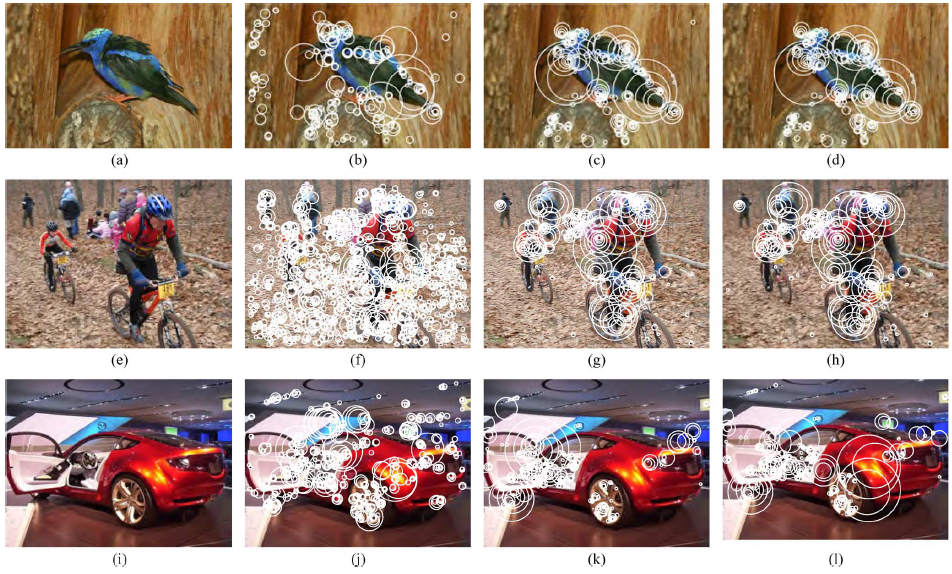• 从图中第1行可以看到论文提及的2个算法得到了非常相近的结果。光照不变点算法忽略了阴影和镜面反射；而颜色增强点忽略了包含常见颜色的模式。两种方法都非常有效的减少了非鲁棒特征的数量。
• 图中第2行的图片有很多的阴影，可以看到应用颜色增强点的图(h)相比应用光照不变点的图(g)鲁棒性更低，而且提取出了更多的模糊特征；而应用$Harris–Laplacian$算法的图(f)则几乎提取了所有的背景阴影。
• 图中第3行则着重展示算法在减少由镜面反射效果产生的特征的能力。应用$Harris–Laplacian$算法的图(j)几乎提取了所有的高光点；而应用光照不变点的图(k)将这些点全部忽略了，减少了数量，而且获得了更稳定的特征。而应用颜色增强点的图(l)着重于检测显著颜色，所以提取了少部分反射点。

## IV 实验

在这部分，作者为了说明论文提及的算法的有效性，分别进行了可重现性实验，图像检索实验以及物体分类实验。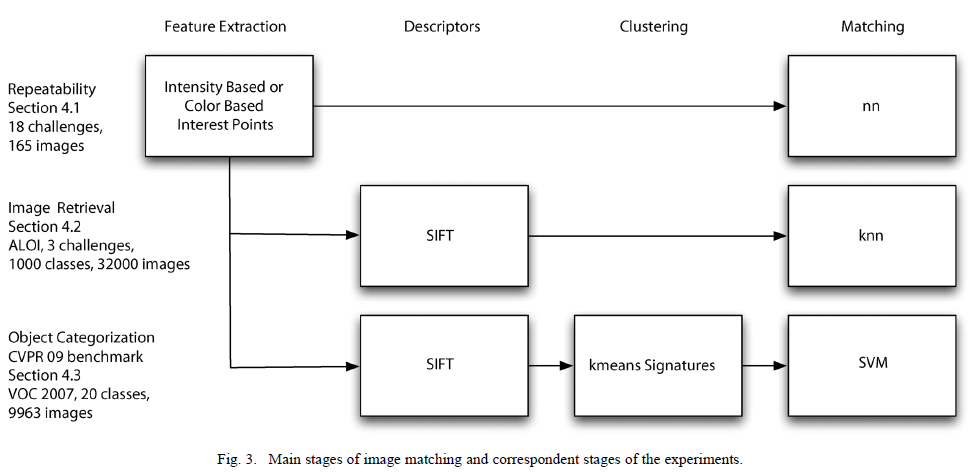### A.可重现性实验（Repeatability）

作者使用了18组带有颜色信息的挑战。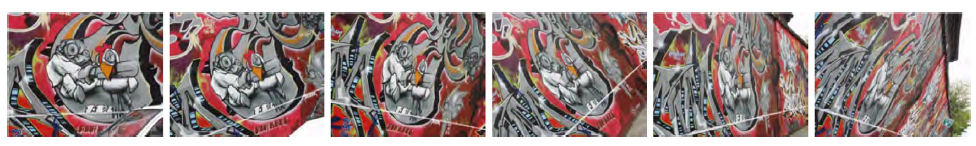Fig.4 用于视角变换的重现性挑战的“Graffiti” 测试集。

### B.图像检索实验（Image Retrieval）

这部分作者使用了ALOI（The Amsterdam Library of Object Images）数据库中的图片进行实验。ALOI包含有大量的人工指定特征的图片。如下图：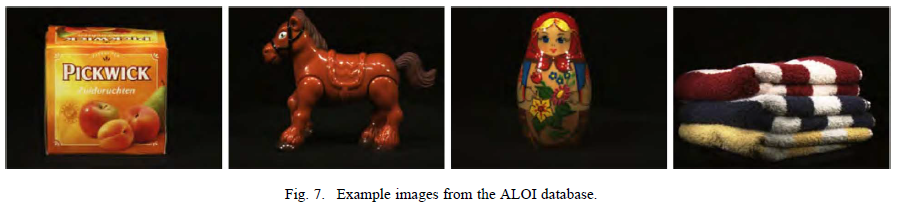### C.物体分类实验（Object Categorization）

这部分作者使用了PASCAL VOC 2007 data set中物体识别部分进行实验。这个数据集中有9963张图片，人工标注出20个物体类别。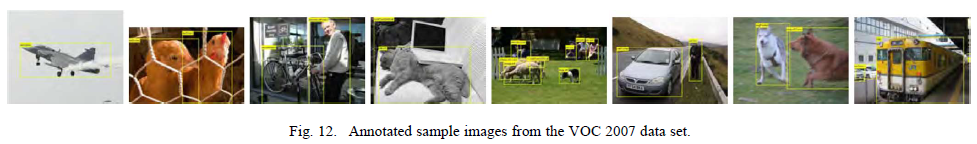以上3组实验的结果都表明论文中的基于颜色的兴趣点检测不仅功能良好，同时又相比传统方法提高了很多的效率。

## 读后感

这是我第一次阅读英文论文，而且相关的技术对我来说几乎是完全陌生的。其中我着重阅读的是算法的实现部分，实验部分的数据分析方面只是泛读。因为平时看过一些英文的教材，所以大大低估了阅读本文的实际困难程度。分析原因如下。

### 论文晦涩难读的原因

• 论文相对于教材，并没有完整的技术进阶曲线
论文不会循序渐进的讲解一个技术，其中很多概念需要读者自己去学习，如果不会的话。
• 论文中的算法描述是不完整的
只是描述了算法的主要内容，或者说与传统方法对比的不同之处，并不能根据文中的描述完整的实现算法。
• 论文中的写作方式并不会像大部分书籍作者那样仔细推敲
文中很多可能会带来歧义的复杂从句，甚至有些病句。

### 阅读的收益

• 翻译并记录了算法实现部分的主要内容，今后进一步学习后会有用处
• 建立了通过阅读论文学习的信心
• 对机器视觉产生了兴趣
• 认识到还要进一步学习数学
• 认识到自己的英文没有想象中那么够用

2016M8009073008• 私有
• 公开
• 删除